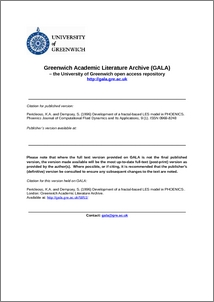# Development of a fractal-based LES model in PHOENICS

and Dempsey, S. (1996) Development of a fractal-based LES model in PHOENICS. Phoenics Journal of Computational Fluid Dynamics and Its Applications, 9 (1). ISSN 0969-8248Preview
PDF (Author Accepted Manuscript)
5951_PERICLEOUS_Fractal-based_LES_Model_in_Phoenics_1996.pdf - Accepted Version

## Abstract

This study concerns the development and validation of a new turbulence model for CFD simulations. The fractal theory of Mandlebrot (1974) and the dissipation-in-a-box formulation of Shreenivasan (1984) are used to determine local dissipation rates for use in a Large-Eddy-Simulation (LES) framework. Such a model has theoretical advantages over “industry standard” two-equation models such as the k-e, (a) because it removes some of the ambiguities associated with the formulation of the e – turbulence energy dissipation equation and (b) it does not assume isotropy above the sub-grid dimension. The model is in fact simpler and numerically more stable that Reynolds stress closures and therefore more useful for engineering computations. The LES model of Ciofallo (1988) is attached to PHOENICS together with the fractal subgrid formulation given here, to create the FLES model.

Item Type: Article turbulence modelling, fractal mathematics School of Computing & Mathematical Sciences > Centre for Numerical Modelling & Process Analysis > Computational Science & Engineering Group 02 Mar 2019 15:52 http://gala.gre.ac.uk/id/eprint/5951View Item